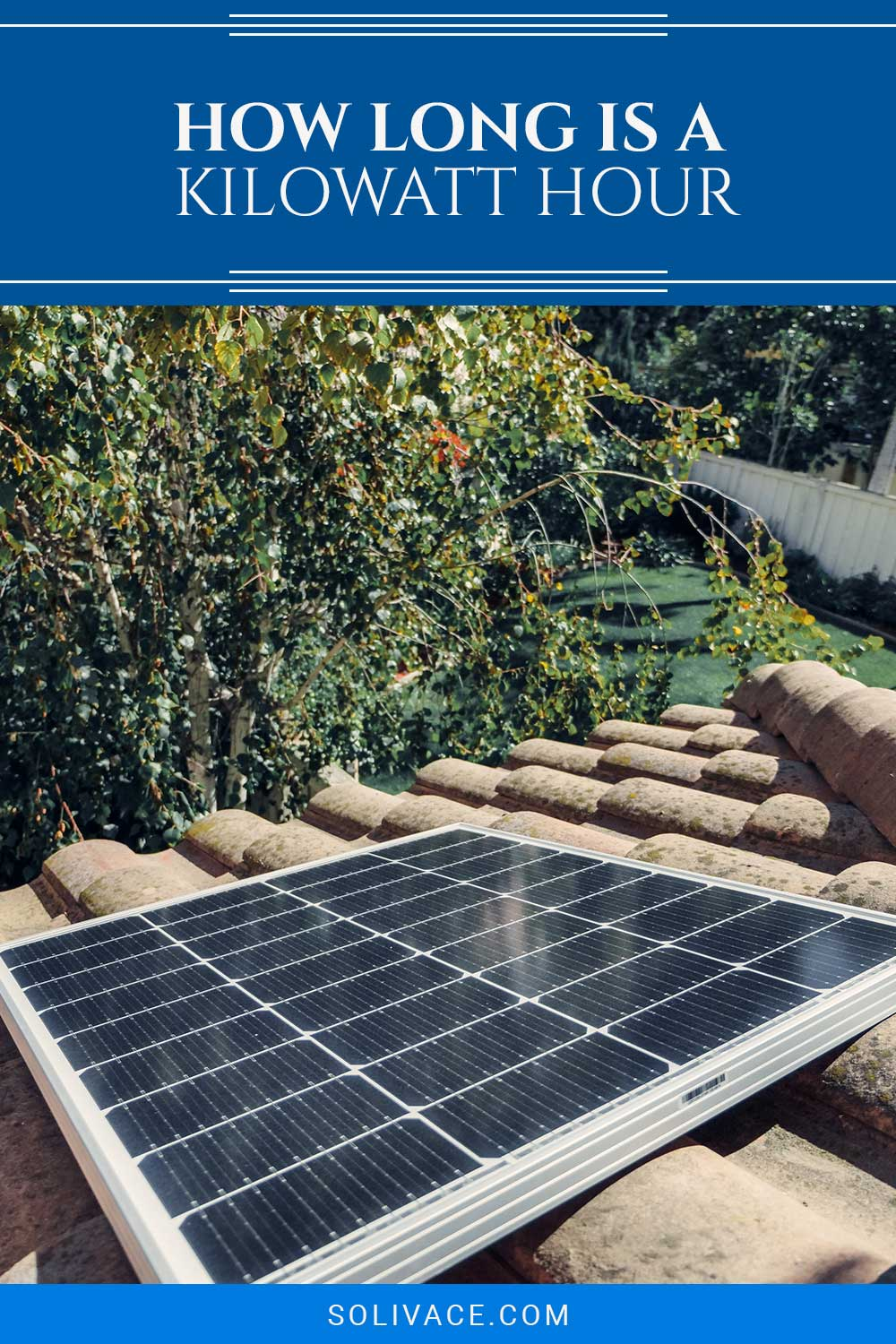# How Long Is A Kilowatt Hour

Last Updated on July 11th, 2023

When it comes to electricity, whether you’re looking at your bills or interested in planning a solar panel system, all the terms and units can be confusing, almost to the point of being purposely obtuse and unhelpful.

A kilowatt-hour (shortened to kWh) is simply a way to measure how much energy is being used by an appliance or household. Specifically, a kWh is the amount of energy used by a 1kW device in an hour.## What Does it Mean?

A kilowatt-hour is simply a unit used to measure how much energy is consumed over time. You might think that because kilowatt-hours have the prefix “kilo-,” the standard unit of energy consumption is watt-hours, but that isn’t the case.

If you were to use watt-hours to represent energy consumption, the numbers would quickly become too large to easily convey meaningful information. The same is true if you were to try to convert the unit to something like kilowatt-minutes.

Put simply, 1kWh is how much time something would take to use 1kW of energy.

## What Does it Represent?

Kilowatt-hours represents a unit of time more than a unit of power. They are not the same as kilowatts which are purely a measurement of power. Measuring the time and power in a single unit gives us an easier idea of energy consumption.

Let’s think about a scenario where we only have 1kWh of energy to use in a survival situation. Using a 100-watt (W) flashlight for 10 hours is probably more useful than a 2,000-watt hair dryer or some other handheld appliance for just half an hour, right?

The thing is that they use the same amount of kilowatt-hours despite having different powers and times.

## What Kilowatt-Hour of Electrical Energy is the Same as?

We already know that kilowatt-hours are not the same as kilowatts, so what are other kilowatt-hour equivalents?

Earlier, we insinuated that kilowatt-hours could not be substituted with watt-hours. This isn’t wholly correct. 1000Wh is, indeed, the same as 1kWh. However, larger numbers quickly become unwieldy to deal with, which is why kilowatt-hours are the standard units.

Similarly, 1kWh is the same as 0.001 megawatt-hours (MWh). Again, these units are useful for particularly large amounts of energy consumption.

Of course, you could convert kilowatt-hours into other units like joules or British Thermal Units (BTU), but they are as useful when it comes to electricity.

## How Many kWh Does a House Use?

The average American household uses about 30kWh per day. This figure assumes a couple of things as an average:

• The household is a three-bedroom house
• The household is comprised of 4 people (typically two parents and two children)
• The household only uses electricity in moderation (they are neither energy wasters nor super-efficient energy savers)

30 kWh a day may be difficult and abstract to think about, so a larger estimation is usually used.

By simply multiplying 30kWh by the number of days in a year, we can get the average amount a typical American home consumes annually. This turns out to be just under 11,000kWh a year.

## What Can you Power with 1 kWh?

A single kilowatt-hour is not all that powerful when you think of 30kWh being used daily. Unfortunately, this means that there aren’t many useful things you can run fully on this small amount of energy.

Here’s an idea of what things you can do with 1kWh:

• Heat 21 premade burritos in a microwave
• Make 160 slices of toast in a toaster
• Enjoy 20 hours of breeze from a ceiling fan
• Watch about 60 hours worth of TV from an energy-efficient 50” LED TV

Keep in mind that if you try to do all of the above (or a combination of your favorite electricity-consuming things) at the same time, you’re looking at much less time for each activity individually and as a whole.

## Final Thoughts on How Long Is A Kilowatt Hour

Kilowatt-hours are just a unit for measuring how much electrical power is consumed within a given time (in this case, an hour). If you’re curious about kilowatt-hours, you may be trying to reduce your energy usage in an attempt to cut down on bills.

While there are many claims of ways to reduce power usage, the only tried and true methods are to switch to more energy-efficient appliances and to unplug electricity, decreasing your usage in a kind of abstinence.